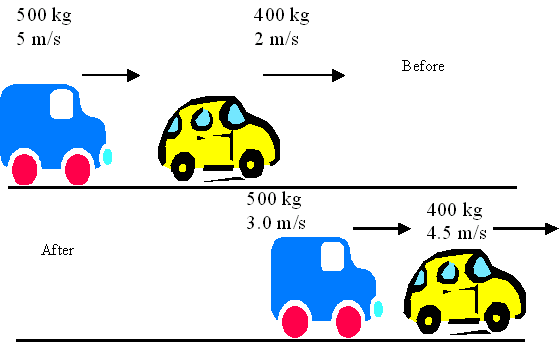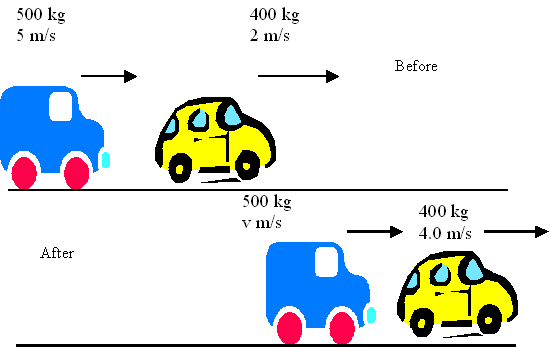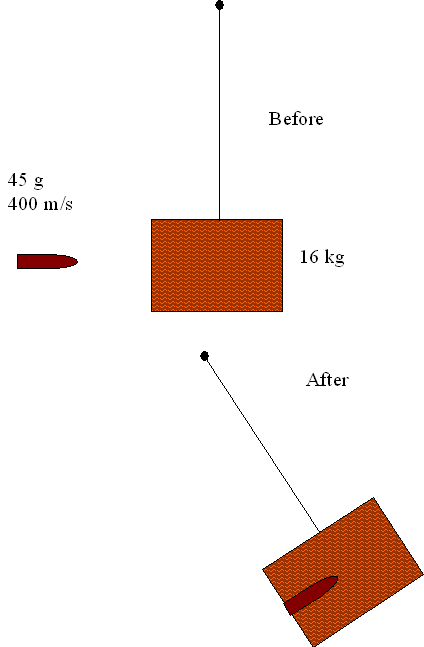# Contoh Soal Exercise Momentum

Exercise Momentum

Answer the questions

1. What is the value of the momentum of a 10 kg ball running down a bowling alley at a speed of 5 m/s?

ANSWER

2. Can you show that the change in momentum is +2mv?

ANSWER

3. The ball has a mass of 200 g, and the value of its velocity throughout remains 6 m/s. What is the change in momentum?

4. The diagram shows two cars at a fairground, before and after bumping into each other. One car and driver has a total mass of 500 kg, while the other car and driver has a total mass of 400 kg.(a) What is (i) the total kinetic energy before the collision;

(ii) the total kinetic energy after the collision.

(iii) the total loss in kinetic energy.

(b) Is this an elastic collision? Explain your answer. ANSWER

5. A second collision is shown below:What is the speed of the 500 kg car after the collision? ANSWER

6. A bullet of mass 45 g is travelling horizontally at 400 m/s when it strikes a wooden block of mass 16 kg suspended on a string so that it can swing freely. The bullet is embedded in the block.Calculate:

a)      The velocity at which the block begins to swing;

b)      The height to which the block rises above its initial position;

c)      How much of the bullets kinetic energy is converted to internal energy.ANSWER

Impulse

7. Can you explain how the formula F = p/t is consistent with Newton II?  ANSWER

8. A car is involved in a collision in which it is brought to a standstill from a speed of 24 m/s. The driver of mass 85 kg is brought to rest by his seat belt in a time of 400 ms.

a)      Calculate the average force exerted on the driver by his seat belt.

b)      Compare this force to his weight and hence work out the g-force

c)   Comment on the likelihood of serious injury.ANSWER

Now try Topic 7 Test

Presentations: Momentum